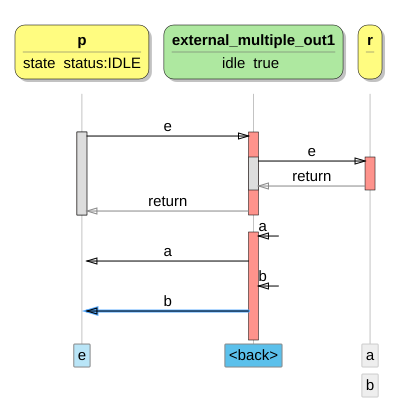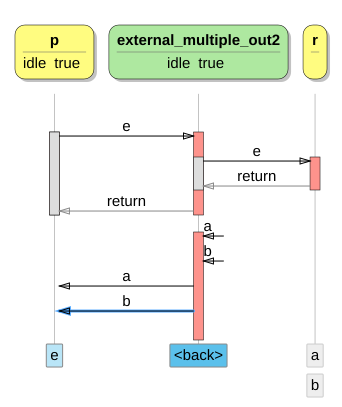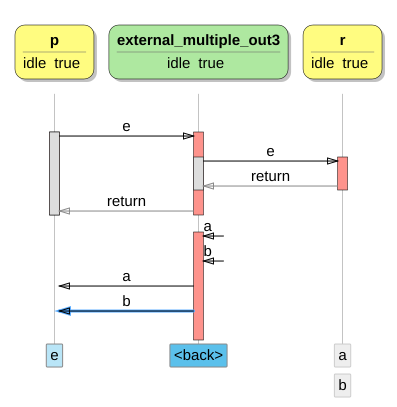### 5.8 External multiple out events

The addition of `external` on a `requires` interface removes the atomicity of an action list, i.e: `{a; b;}`. Also see External.

The first example shows how the behavior of `external` `J1` interface transforms into the interface behavior of `I1` by forwarding the events in the `external_multiple_out1` component behavior.

```interface I1
{
in void e ();
out void a ();
out void b ();
behavior
{
enum status {IDLE, A, B};
status state = status.IDLE;
[state.IDLE] on e: state = status.A;
[!state.IDLE] on e: illegal;
[state.A] on inevitable: {state = status.B; a;}
[state.B] on inevitable: {state = status.IDLE; b;}
}
}

interface J1
{
in void e ();
out void a ();
out void b ();
behavior
{
on e: {a; b;}
}
}

component external_multiple_out1
{
provides I1 p;
requires external J1 r;
behavior
{
bool idle = true;
[idle] on p.e (): {idle = false; r.e ();}
[!idle] on p.e: illegal;
on r.a (): p.a ();
on r.b (): {idle = true; p.b ();}
}
}
```Two variations of the model above can be considered. Both variants provide the same interface behavior (`I2` and `I3` are identical), but differ in their requires interface behavior and as a result in their component behavior.

The first variant uses the requires behavior (`J1` and `J2` are identical) as the first example. The component takes care of joining the independently received events `a` and `b` as required by its provides interface.

```interface I2
{
in void e ();
out void a ();
out void b ();
behavior
{
bool idle = true;
[idle] on e: idle = false;
[!idle] on inevitable: {idle = true; a; b;}
}
}

interface J2
{
in void e ();
out void a ();
out void b ();
behavior
{
on e: {a; b;}
}
}

component external_multiple_out2
{
provides I2 p;
requires external J2 r;
behavior
{
bool idle = true;
[idle] on p.e (): {idle = false; r.e ();}
[!idle] on p.e: illegal;
on r.a (): {}
on r.b (): {idle = true; p.a (); p.b ();}
}
}
```This variation provides the same interface as it requires. The component however, must make sure to join `a` and `b` again to implement its provides interface behavior.

```interface I3
{
in void e ();
out void a ();
out void b ();
behavior
{
bool idle = true;
[idle] on e: idle = false;
[!idle] on e: illegal;
[!idle] on inevitable: {idle = true; a; b;}
}
}

component external_multiple_out3
{
provides I3 p;
requires external I3 r;
behavior
{
bool idle = true;
[idle] on p.e (): {idle = false; r.e ();}
[!idle] on p.e: illegal;
on r.a (): {}
on r.b (): {idle = true; p.a (); p.b ();}
}
}
```